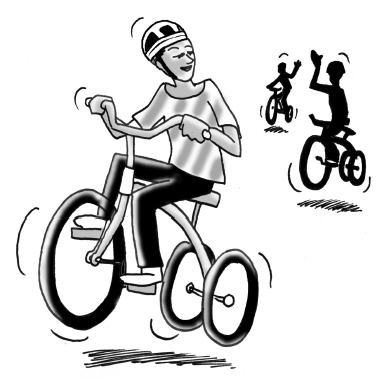### Home > AC > Chapter 7 > Lesson 7.2.2 > Problem7-65

7-65.

THE BIG RACE − HEAT 2Barbara, Elizabeth, and Carlos participated in the second heat of “The Big Race.” Barbara thought she could win with a $3\text{-meter}$ head start even though she only pedaled $3$ meters every $2$ seconds. Elizabeth began at the starting line and finished the $20\text{-meter}$ race in $5$ seconds. Meanwhile, Carlos rode his tricycle so that his distance $\left(y\right)$ from the starting line in meters could be represented by the rule $y=\frac{5}{2}x+1$, where $x$ represents time in seconds.

1. Using the given information, graph lines for Barbara, Elizabeth, and Carlos on the same set of axes. Who won the $20\text{-meter}$ race and will advance to the final race?

2. Find rules that describe Barbara's and Elizabeth's motion.

What are the slope and y-intercept of each of their lines?

3. How fast did Carlos pedal?

Use the graph. What part of the graph shows his rate? How far does Carlos pedal each second?

After $2$ seconds. Where is this shown on the graph? Use the Equal Values Method to confirm your answer algebraically.